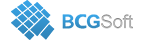BCGControlBar Pro for MFC
 virtual BOOL CBCGPChartBaseFormula::IsNonDiscreteCurve ( ) const
inlinevirtual

Tells whether the formula is displayed as a non-discrete curve.

This method tells whether the formula is displayed as a non-discrete curve. Points of non-discrete curves are calculated for each pixel on the X axis. Points of discrete curves are calculated as regular data points.

Reimplemented in CBCGPChartTrendFormula.